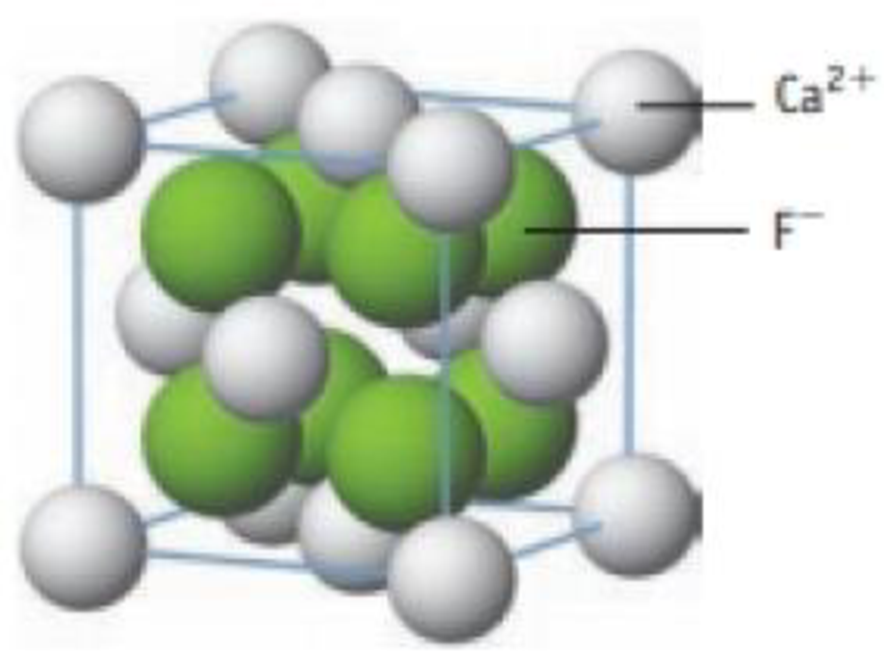Chapter 12, Problem 8PS

Chapter
Section
Textbook Problem

The mineral fluorite, which is composed of calcium ions and fluoride ions, has the unit cell shown here. (a) What type of unit cell is described by the Ca2+ ions? (b) Where are the F− ions located, in octahedral holes or tetrahedral holes? (c) Based on this unit cell, what is the formula of fluorite?Unit cell of fluorite

(a)

Interpretation Introduction

Interpretation:

Type of unit cell described by the Ca2+ atoms has to be identified.

Explanation

The calcium ions form the cubic close packing (face centered cubic) arrangement, because, the calcium ions o...

(b)

Interpretation Introduction

Interpretation:

Arrangement of F ion has to be identified.

(c)

Interpretation Introduction

Interpretation:

Formula for fluorite has to be identified.

Still sussing out bartleby?

Check out a sample textbook solution.

See a sample solution

The Solution to Your Study Problems

Bartleby provides explanations to thousands of textbook problems written by our experts, many with advanced degrees!

Get Started